• ### Miller's Pitch Shifting Example From His Book

I've never really dug into pitch shifting before and I have started looking at Miller's pitch shifting patch example from his book but I'm a little confused by the graph in this related example, specifically Fig 7. 17. (I think just because how the qualifying text is worded). I have a few clarifying questions so hopefully someone can humor me.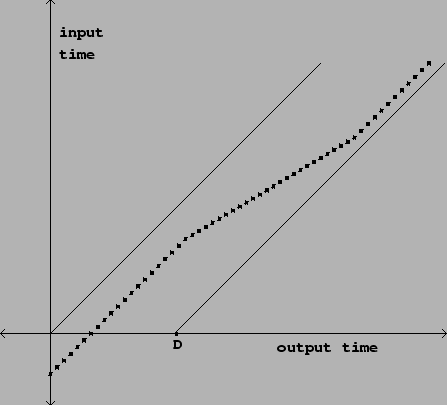1. The diagonal line from origin (0,0) is the input signal; the dotted line is the variable delay line; the diagonal line at D is the delay line over time relative to the input signal, yes?
2. D is the distance from the origin point to the point marked D on the x axis, correct? This is the max length of the delay line?
3. The y axis shows the quantity `n - d[n]` where `d[n]` represents the delay in samples and this is where I get a bit fuzzy: graphically, what is the output sample here? Why am I subtracting? What am I subtracting?

• Posts 21 | Views 1626
• Cool. Thanks, @jameslo.

So, getting to the Pitch Shifter example itself, it's easy enough to follow the transposition factor from the math to patch but I'm wondering why the -1 preceding the tape head rotation frequency isn't the current sample rate, as per the math?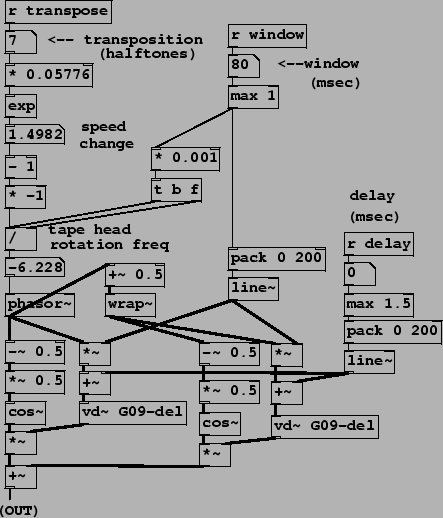`f = (t - 1) * R / s`

I see where one is subtracted from t and where the window size is divided into that but why does R = -1? Is this some other interpretation of 'sample rate?'

• @ricky Having looked for 10 minutes I can't figure out how the formula for f relates to the computation in the sample patch, but just looking at the patch itself, the negative frequency (i.e. multiplication by -1) makes sense to me because you want ever decreasing delay amounts. [phasor~] is an increasing ramp with positive frequencies.

• So, you're saying the `-1` is in place to ensure we get decreasing delay amounts for when we want to pitch up and increasing delay amounts when we want to pitch down because of the nature of phasor~? That makes sense but it doesn't explain the `R` in the math.

• @jameslo - I remembered this live granular synthesis example from the Pd tutorial website which makes sense to me.

The transposition factor is handled as `pow(2, t/12) - 1 * 44100/s)` where t is the transposition step and s is the windows size. Maybe we can reconcile the differences and figure out how Miller factor the sample rate.

• It's a bad idea to post after cocktail hour, but here goes:

Regarding sample rate R, go back to the original transposition formula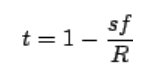Note that R is in samples/second and s is in samples/window. But in the example patch, s is specified in seconds (after the multiplcation by 0.001). So that factors out the sample rate coming from the transposition factor because you'd have to multiply s in seconds by the sample rate to get s in samples.

But that doesn't explain the [* -1]. I think there's just an algebra mistake in the text. Go ahead and solve the original transposition formula for f and you'll see that the sign is flipped.

• Cocktail hour is the best hour. Good man and good catch! I totally forgot about the 0.001 multiplier and of course it factors it out. D'oh. As for the [* -1], I thought this made sense in the context of the Pd patch given the positive ramp of the phasor and without it the pitch transposition step is inverted.

What you're saying is that the algebra below is incorrect? Maybe it has something to do with the way the transposition factor is calculated?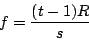It's too early for a cocktail here. Still on my first coffee.

• I'm serious: solve the original transposition formula for f. Show your work• @ricky It's [- 1] because the exponent of 0 (no shift) is 1 but the tape head has to be stationary (no rotation = 0) for playback at normal speed......
And [* -1] because (although it seems wrong until you really think about it) the tape head has to be turning backwards relative to the tape (negative values for [phasor~]...) for the pitch to increase (positive transposition values).
There is no point arguing with the patch because it works as expected.......[cos~] windows the output so that amplitude sums are "sort of" ok (with the in and out of phase bits) and the vertical edge of the [phasor~] saw is declicked.
And of course the change of delay gives the rotating read head effect.

@katjav gave it a lot more thought here........ https://www.katjaas.nl/pitchshift/pitchshift.html
I could be wrong but I don't see why the samplerate would be part of the calculation (it isn't)...... as all variables are relative.
David

• @whale-av said:

@ricky It's [- 1] because the exponent of 0 (no shift) is 1 but the tape head has to be stationary (no rotation = 0) for playback at normal speed......

Ah, thanks. That makes sense. Thanks, David.

And [* -1] because (although it seems wrong until you really think about it) the tape head has to be turning backwards relative to the tape (negative values for [phasor~]...) for the pitch to increase (positive transposition values).
There is no point arguing with the patch because it works as expected.......Who is arguing?This was my understanding.

[cos~] windows the output so that amplitude sums are "sort of" ok (with the in and out of phase bits) and the vertical edge of the [phasor~] saw is declicked.

Yes, a nicely scaled fade in and out.

• @whale-av said:

@katjav gave it a lot more thought here........ https://www.katjaas.nl/pitchshift/pitchshift.html
I could be wrong but I don't see why the samplerate would be part of the calculation (it isn't)...... as all variables are relative.

I was confused because R represents sample rate earlier in the text.

"If the frequency of the sawtooth wave is \$f\$ (in cycles per second), then its value sweeps from 0 to 1 every \$R/f\$ samples (where \$R\$ is the sample rate)."

• @ricky I think that is just to work out a good window size with enough samples in it but not too many,
The relationship between a semitone interval and frequency is a ratio....... discovered long before digital audio when "samplerate" might have referred to wine tasting (a bit before cocktails too........).
As I said I could be wrong but I cannot see anything in the patch and cannot imagine how the samplerate could be relevant to the [phasor~] frequency.
Sorry about the "arguing"..... and thank you for the link. I always thought Millers book was a real paper thing, and didn't know it was online.
David.

• Hmm, it's interesting that these kinds of time-domain algorithms use cosine-shaped windows and 2X overlap, whereas FFT resynthesis algorithms use Hann windows and 4X overlap. I've fooled around a little with FFT resynthesis using the former, and the sound is just a little coarser, which is to say I was surprised it wasn't terrible. I wonder if for something like time-domain pitch shift Hann+4X overlap would sound better (i.e. have softer windowing artifact) and if so, why.

(This question occurs to me just as I'm preparing to have no free time for a week so that's why I'm not just trying it and posting my results with further questions)

• I thought the cosine shape was really just convenience given that [cos~] is available and that allows for a sample-accurate solution that doesn't involve reading from a table.

• For future students & fellow nerds, here’s what I was suggesting: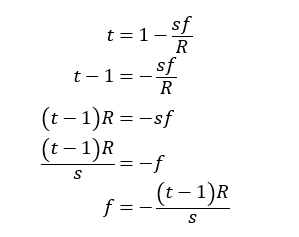See? Opposite sign, contrary to the text.

RE sample rate, let s’ = s/R (in English: specify the window size in seconds instead of samples). Then: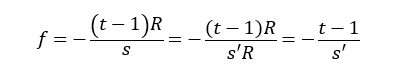which is what the example patch G09 is computing for the phasor frequency.

RE Hann windowing with 4x overlap, it definitely sounds worse in this case, still not sure why when it's better for the FFT.
time domain overlapped windowing.zip
I have a faint memory of an explanation in Miller's book why the positive part of the cosine function is a useful windowing function for time domain stuff, but I can't find it. Maybe I just saw it used a lot in the audio examples.

Posts 21 | Views 1626
Internal error.

Oops! Looks like something went wrong!